# Selina Solutions Concise Maths Class 10 Chapter 24 Measures of Central Tendency

The numerical expressions which represent the characteristics of a group (a large collection of numerical data) are called Measures of Central Tendency. In this chapter, students will learn and solve problems based on three types of statistical averages, namely Mean, Median and Mode. Further in the chapter, different methods of finding these central tendencies are studied and tested under the exercise problems. Students can refer to the Selina Solutions for Class 10 Mathematics for clarifying any doubts regarding solving problems. It also helps students for their exam preparations and mainly improves problem-solving skills, which is vital. The solutions of the Selina Solutions for Class 10 Mathematics Chapter 24 Measures of Central Tendency exercises are available in the links given below.

## Selina Solutions Concise Maths Class 10 Chapter 24 Measures of Central Tendency Download PDF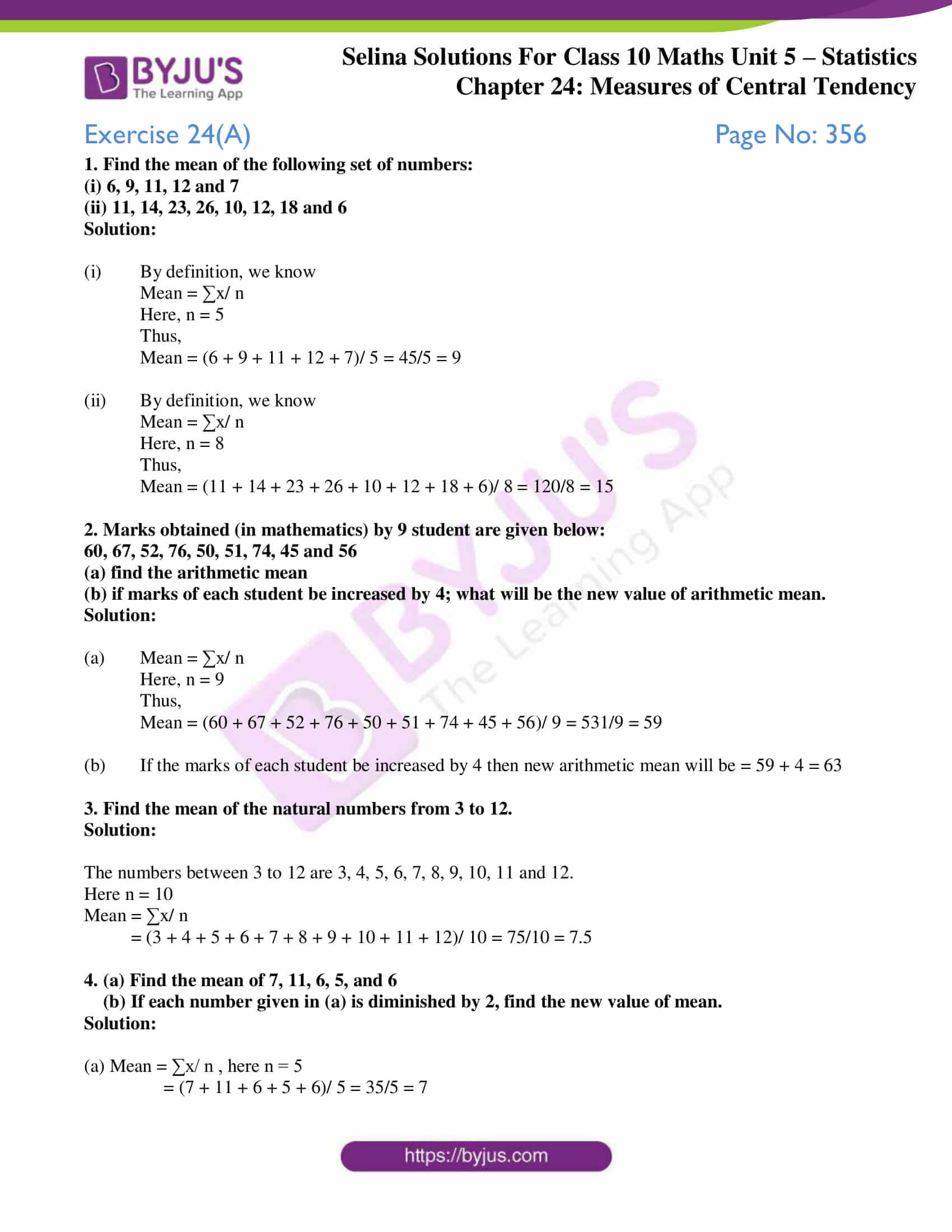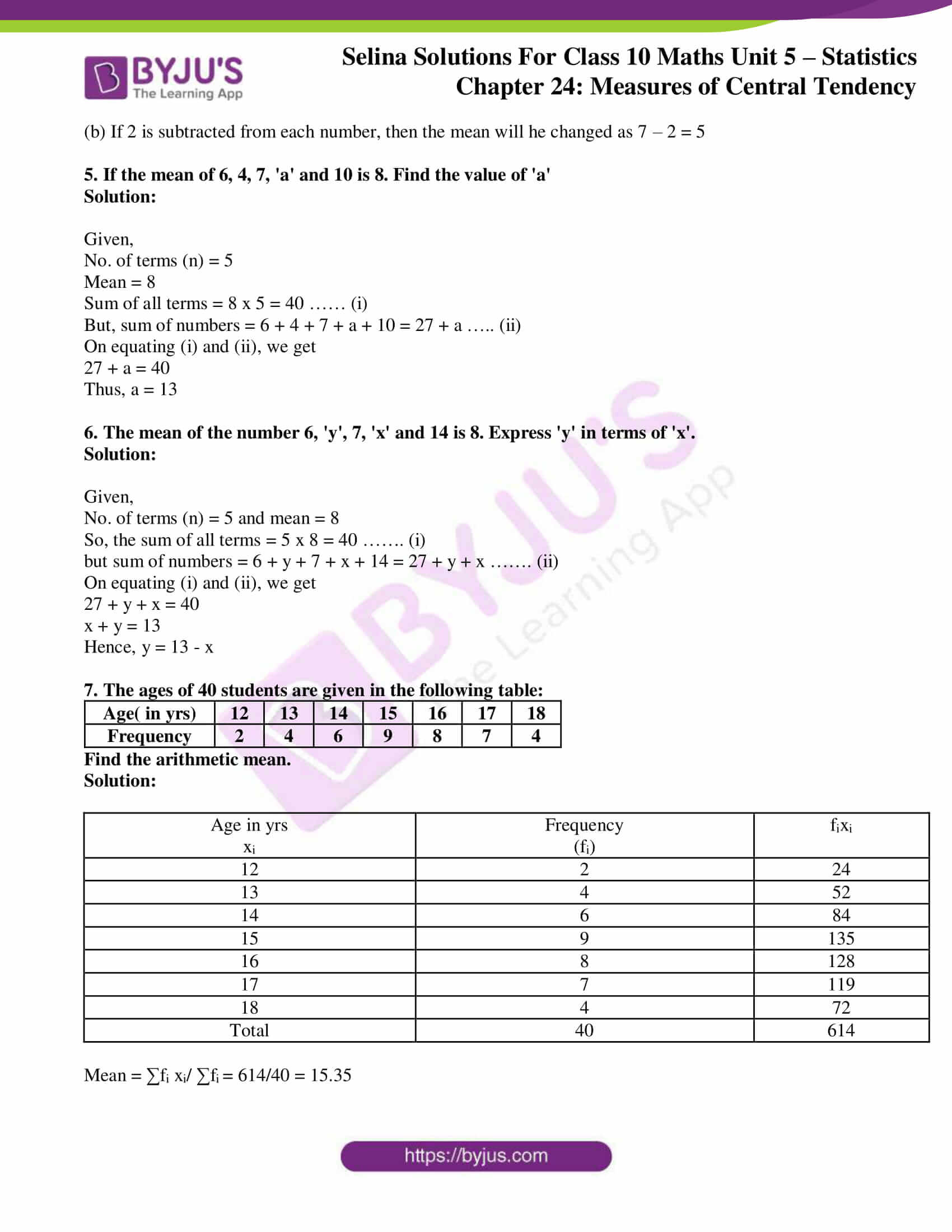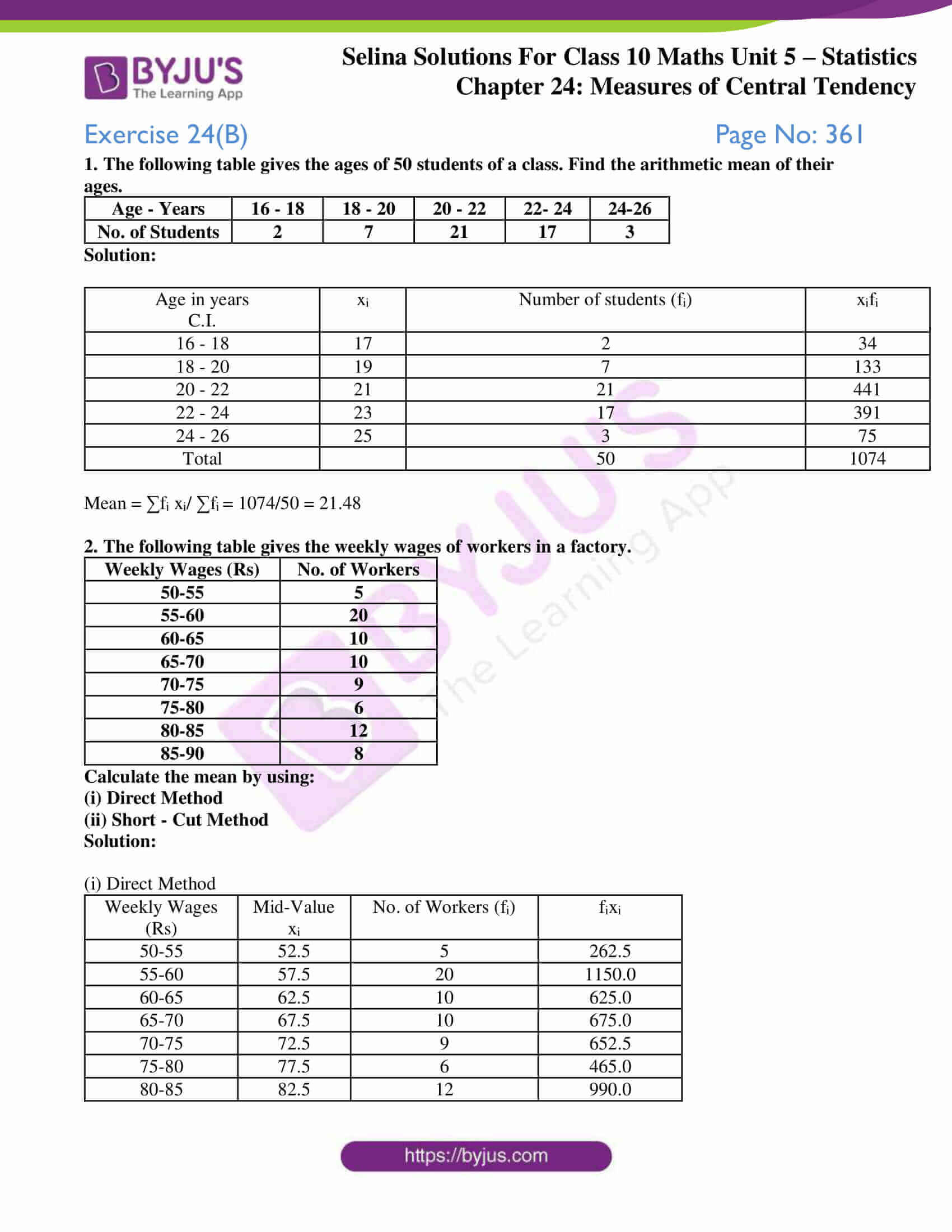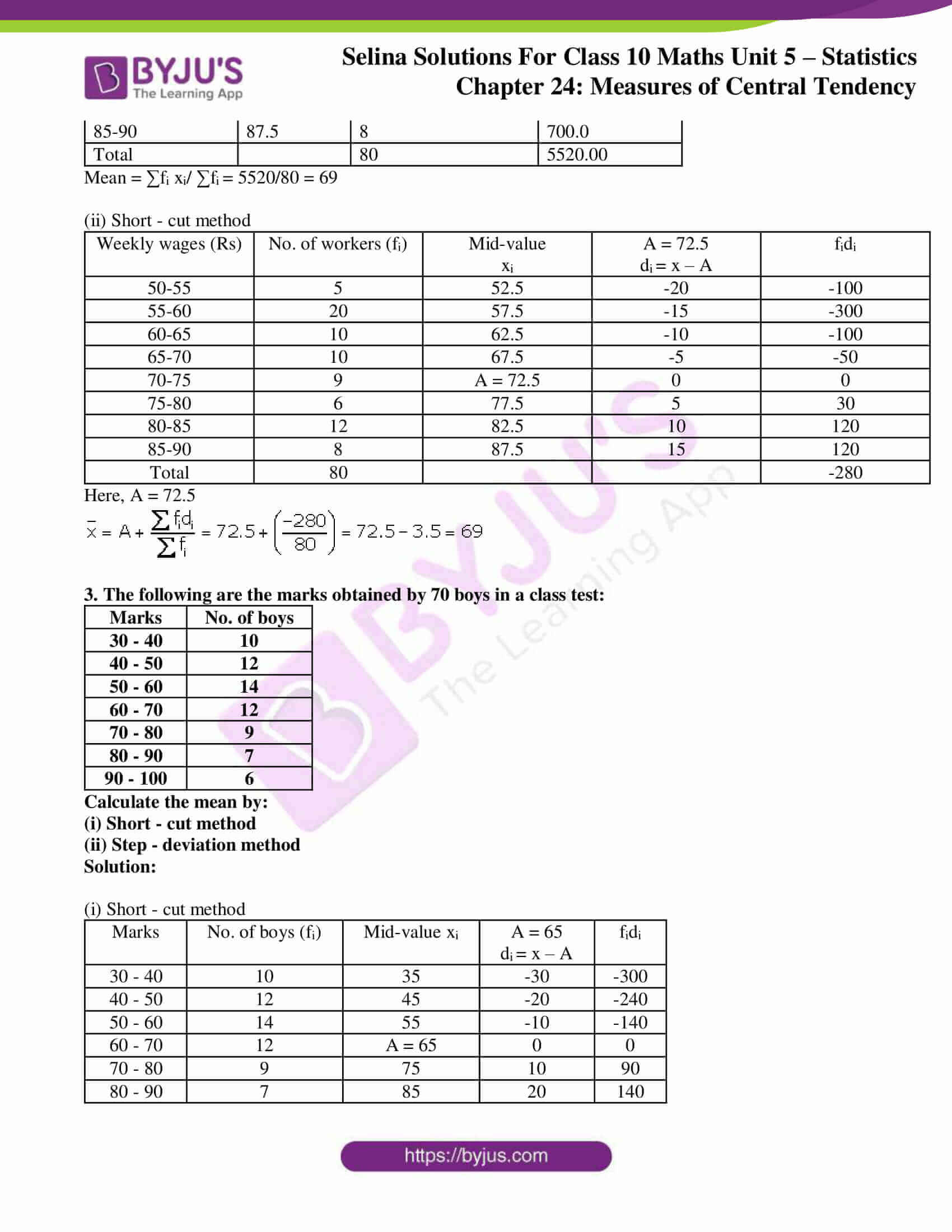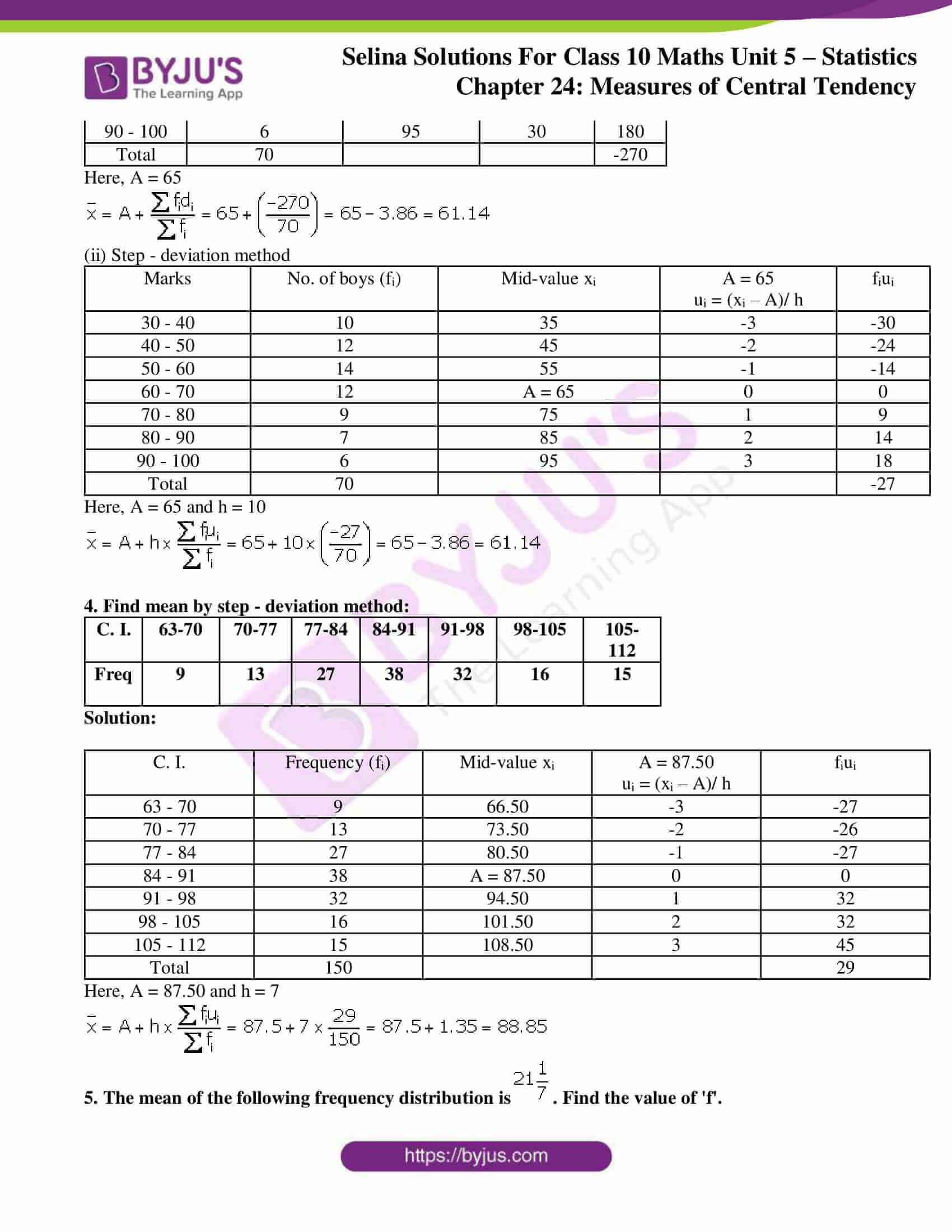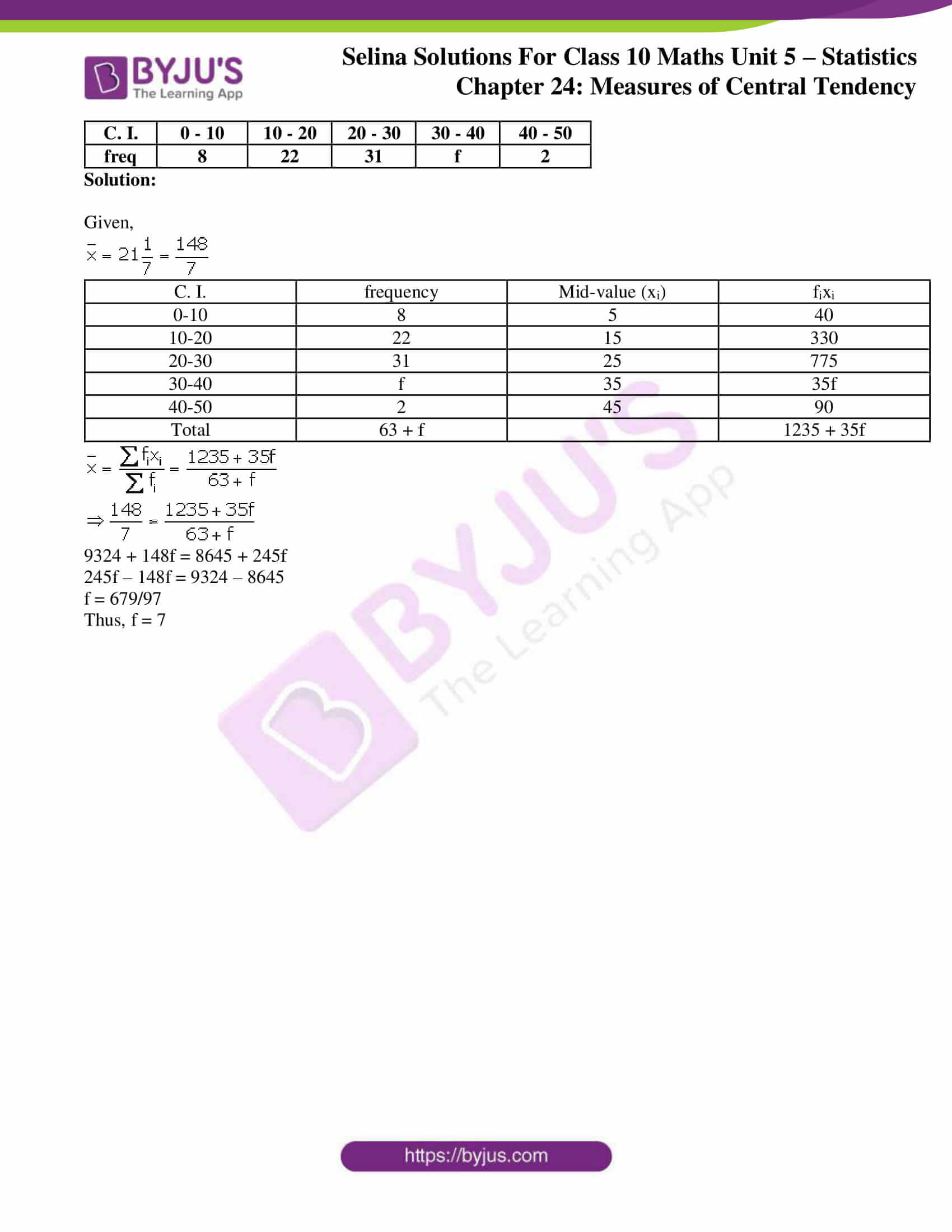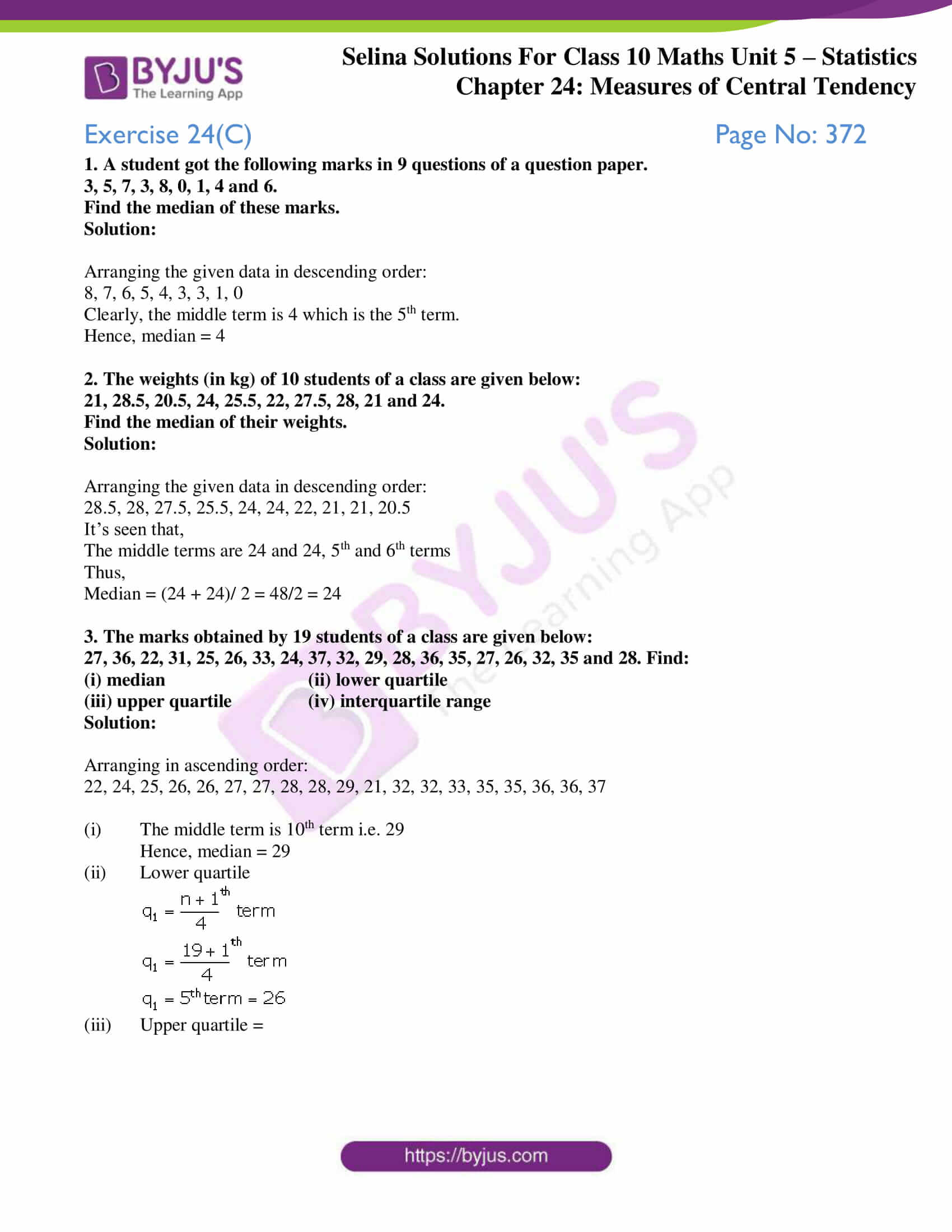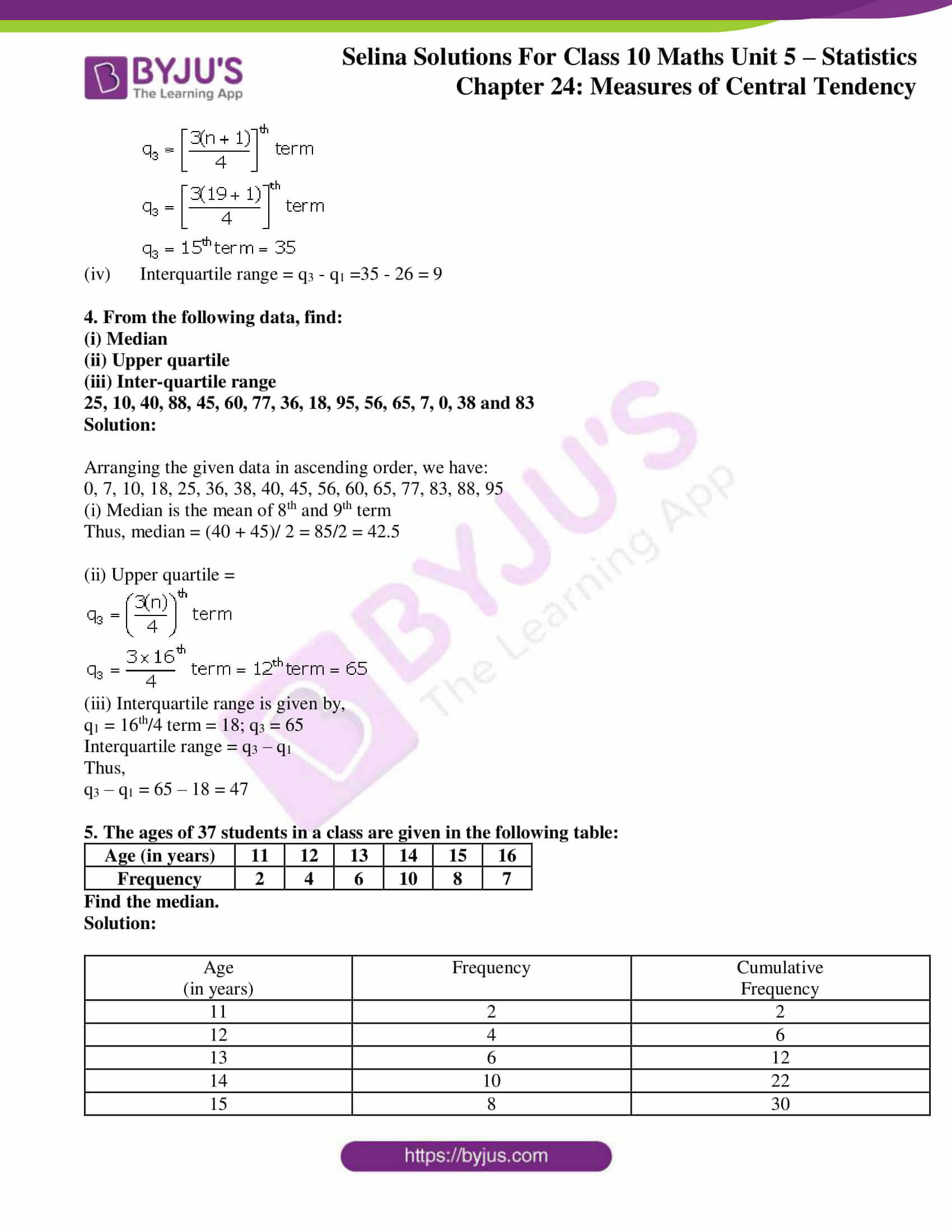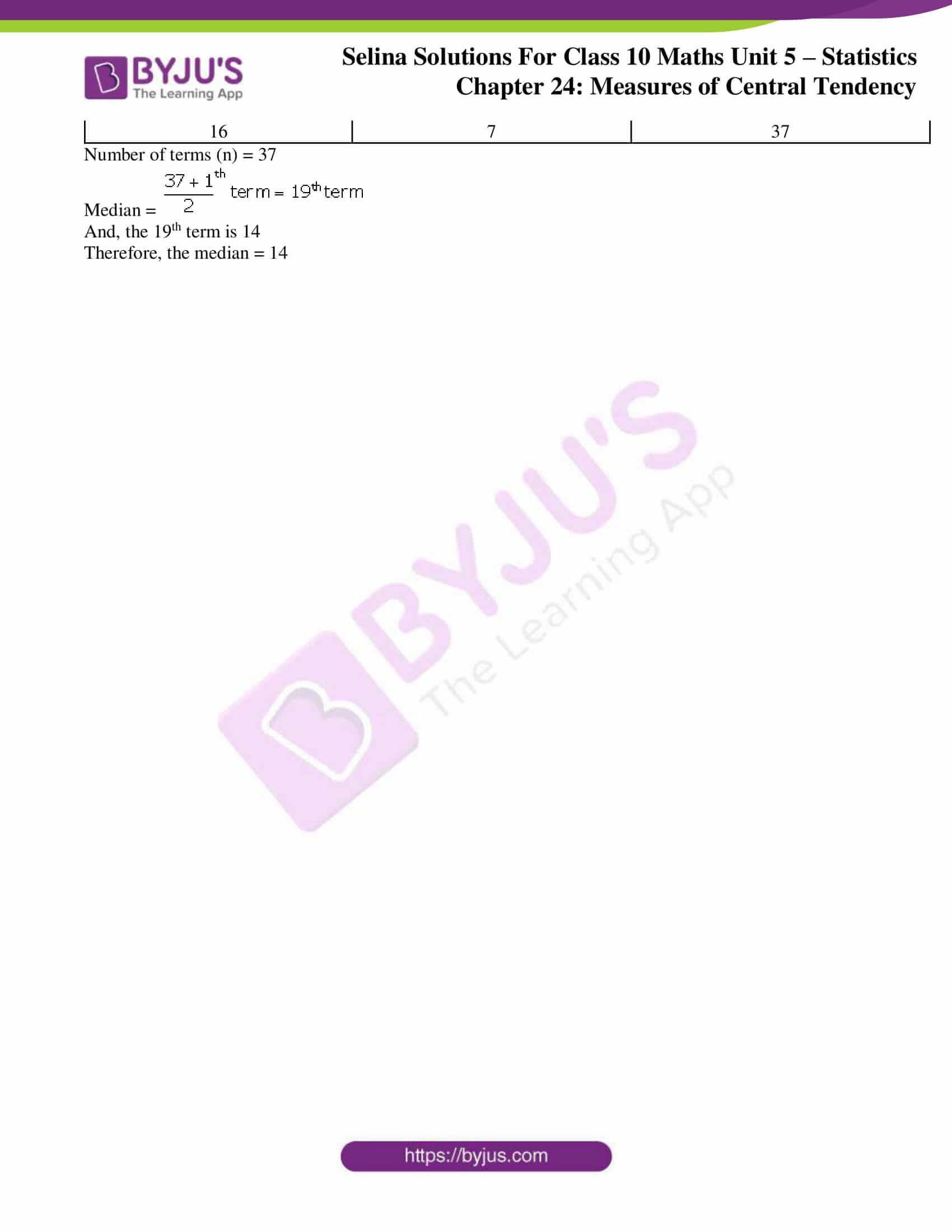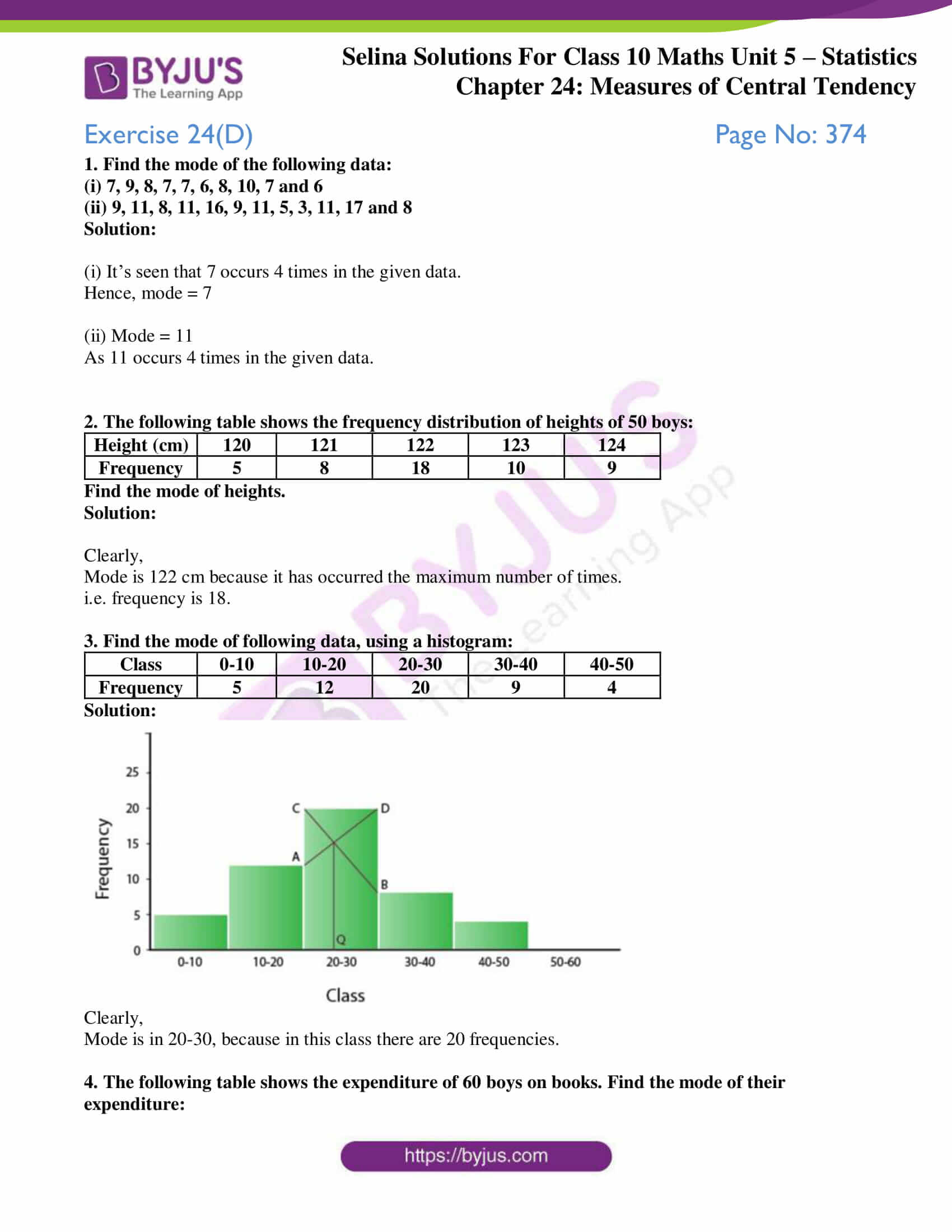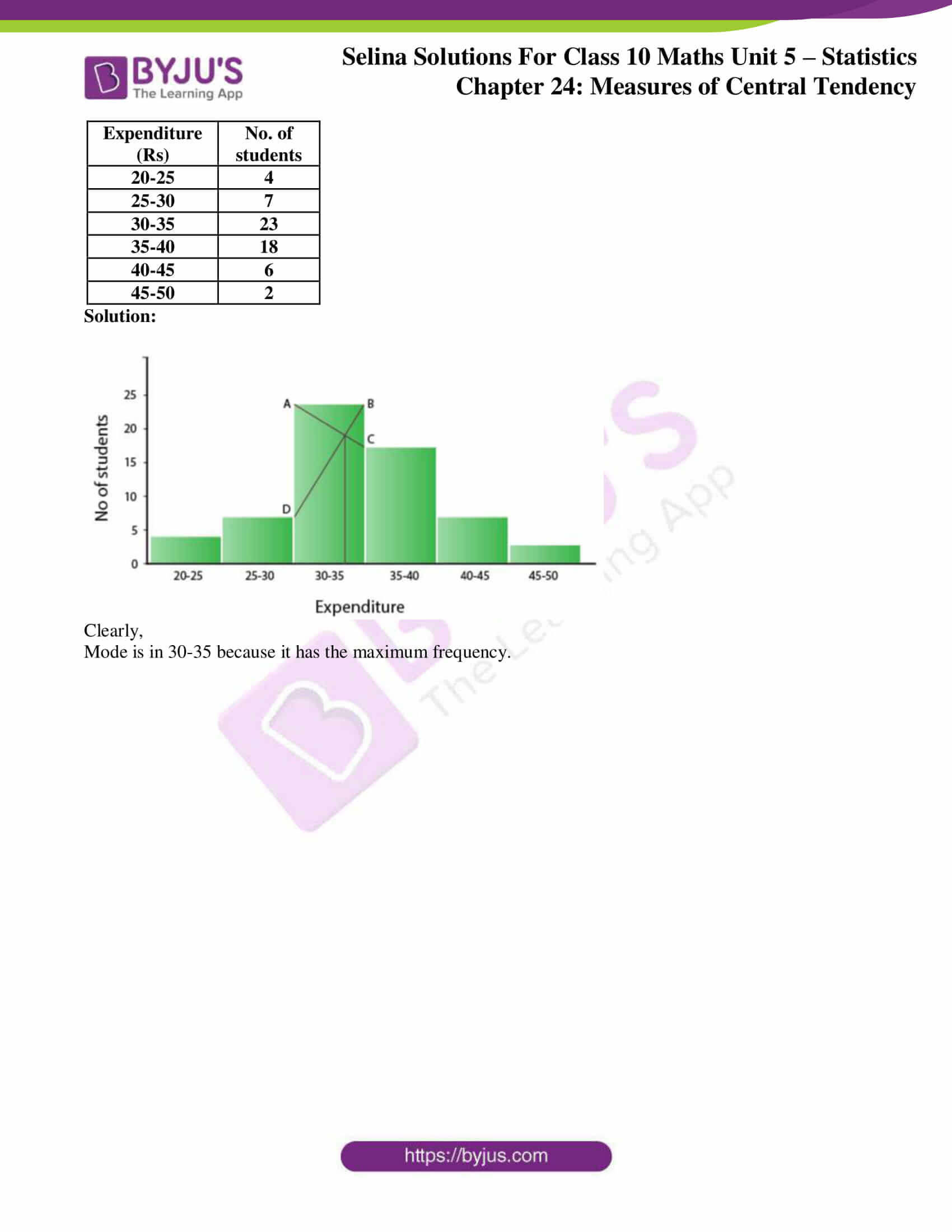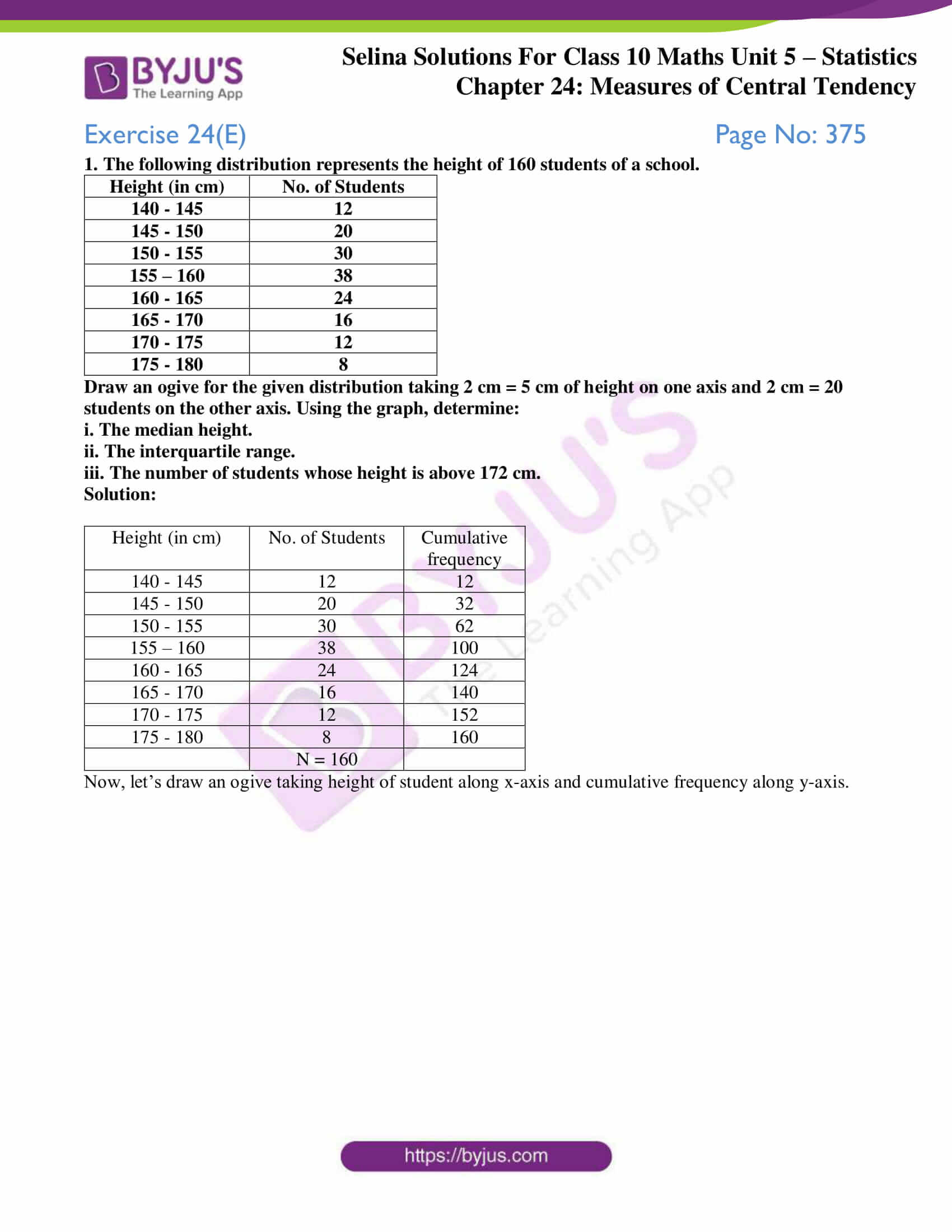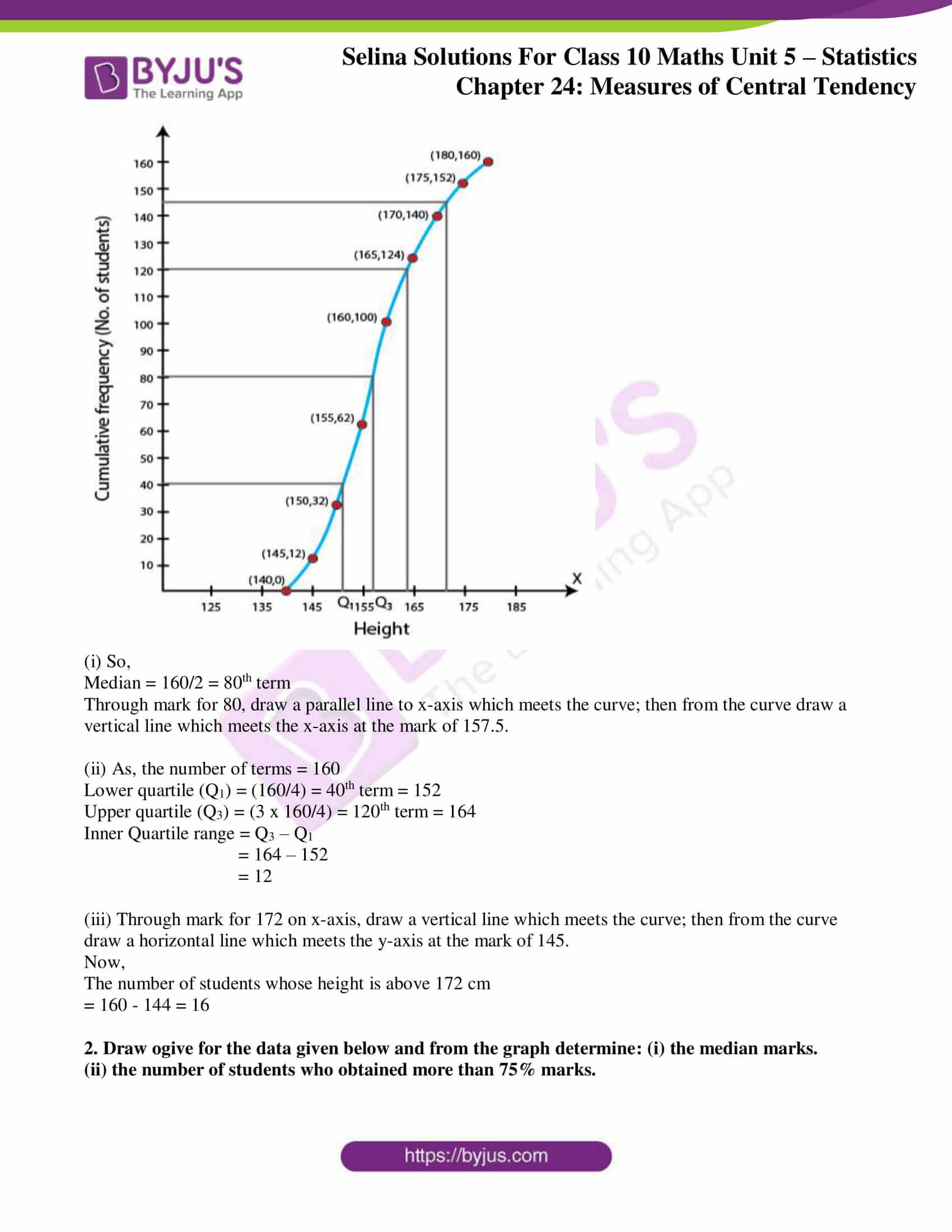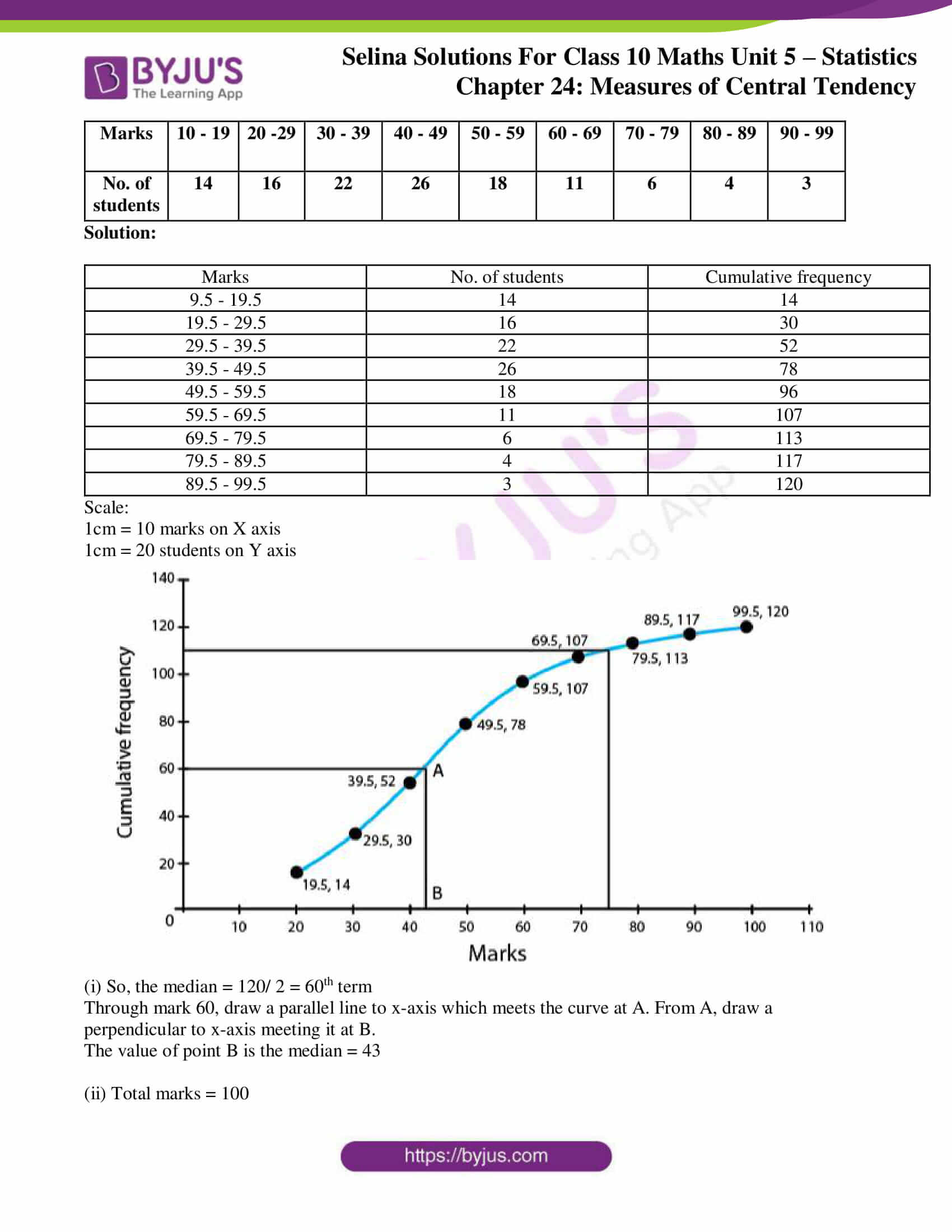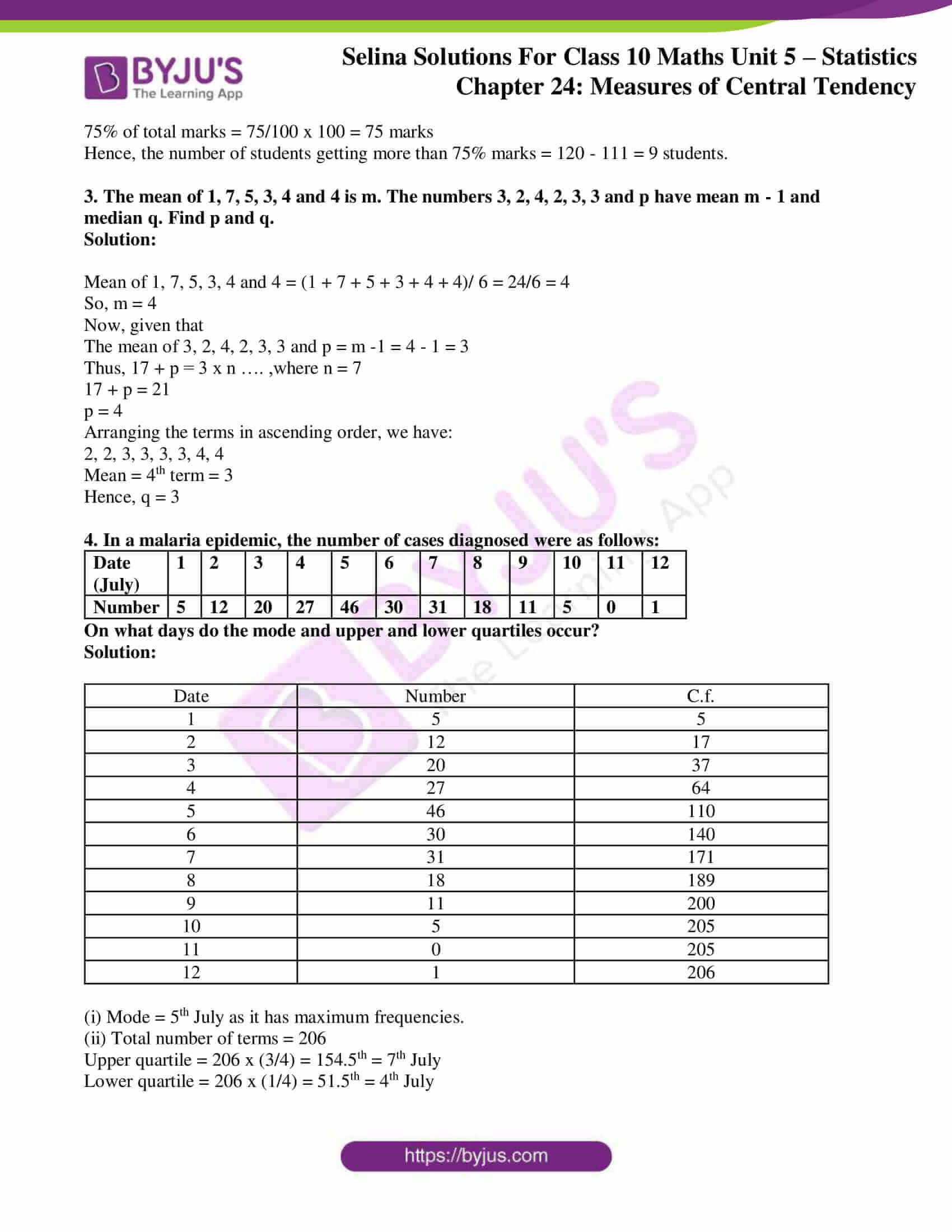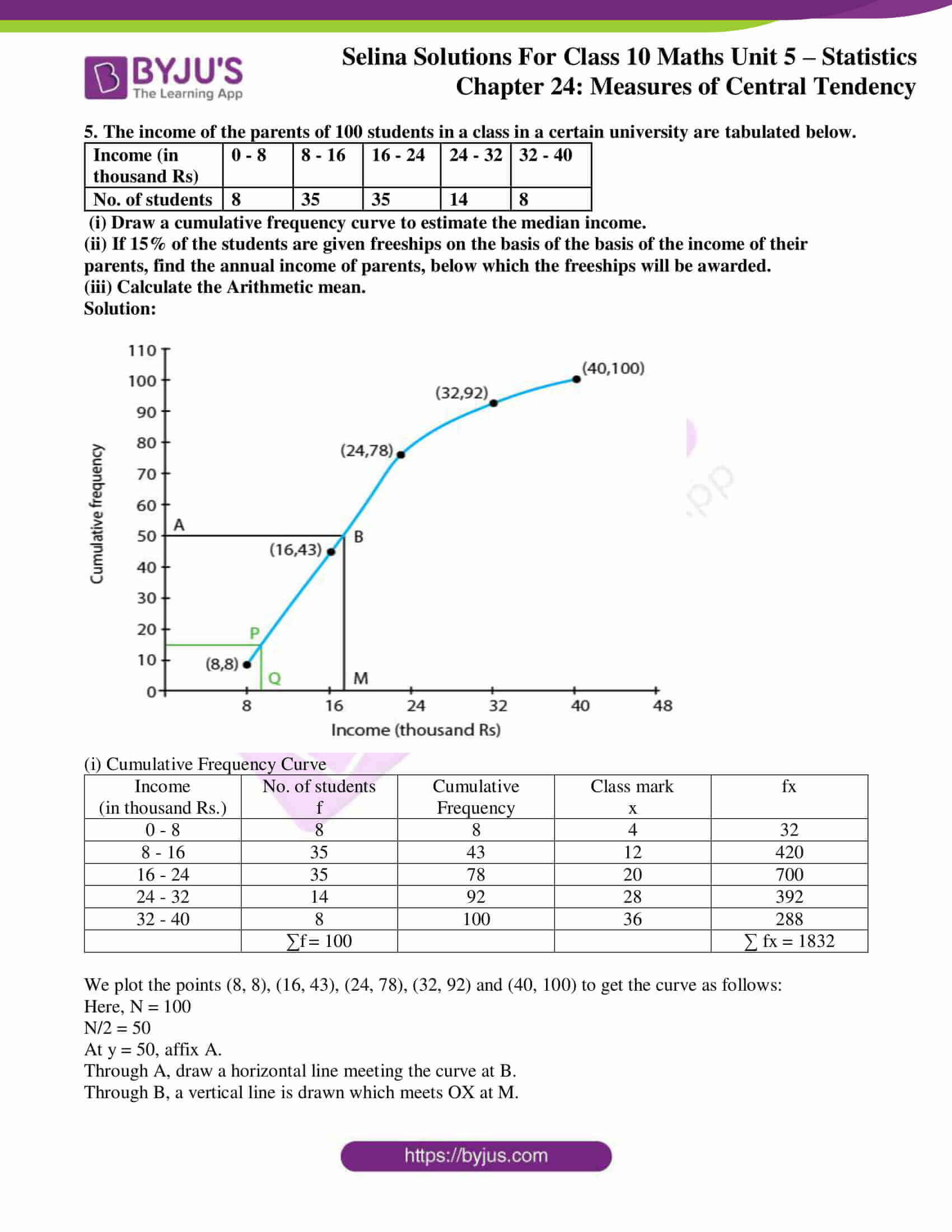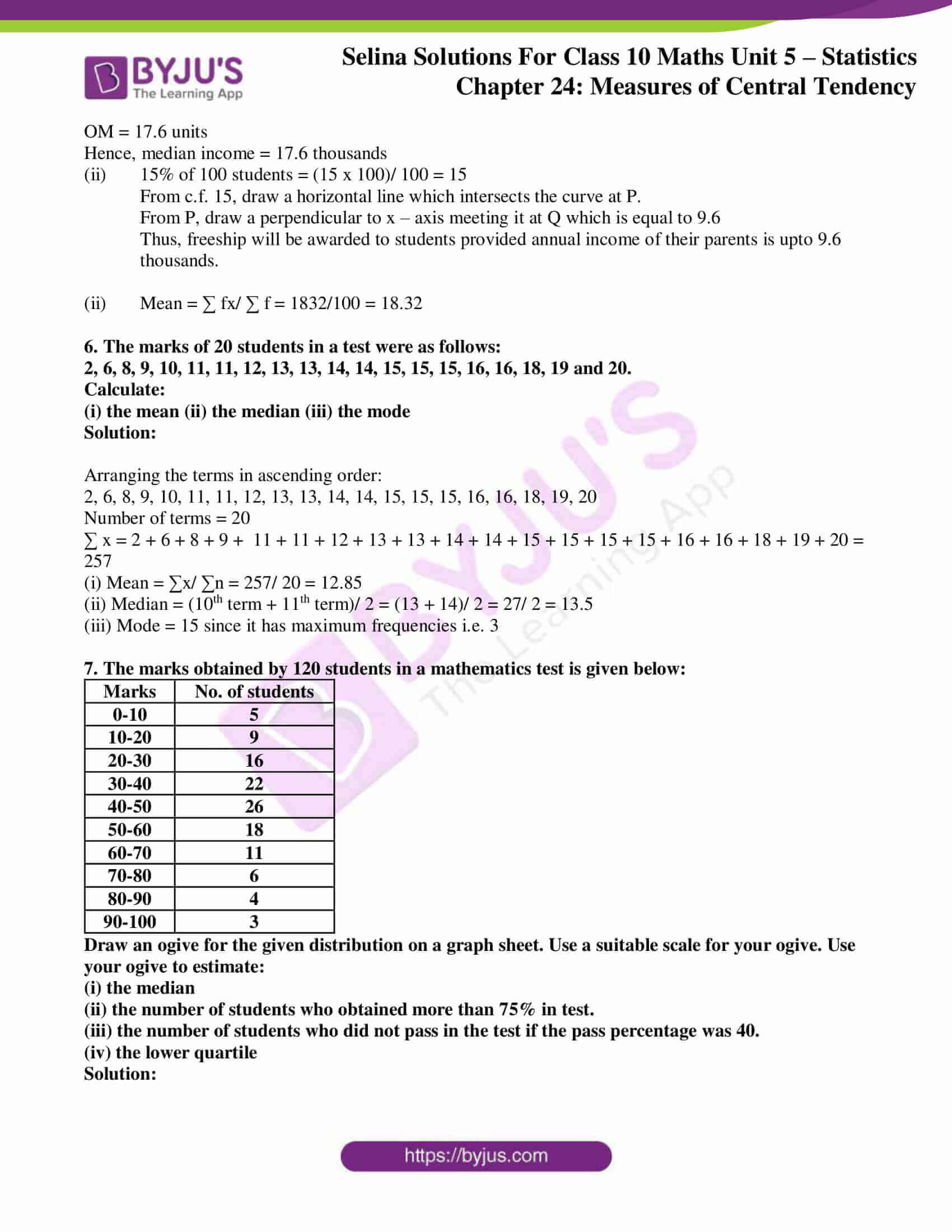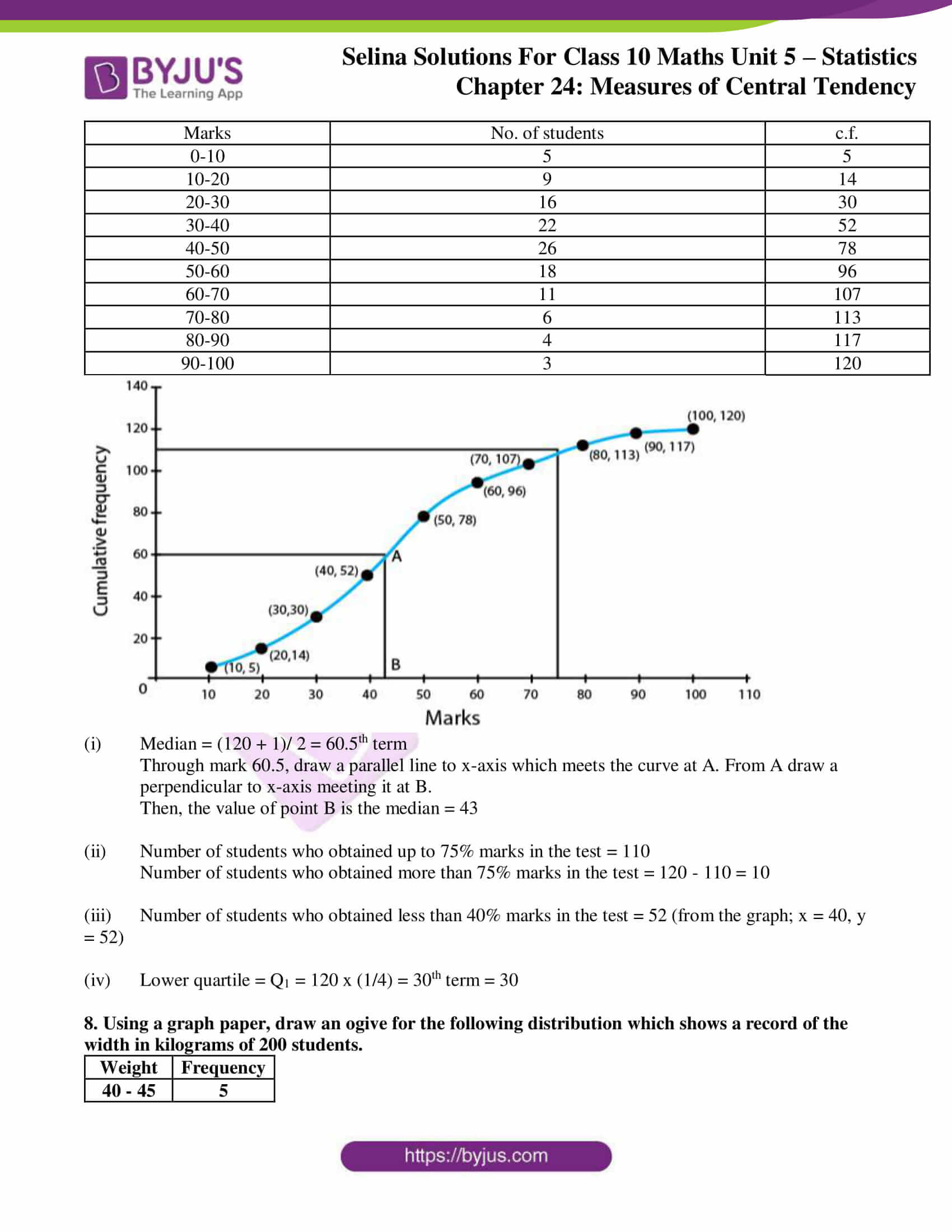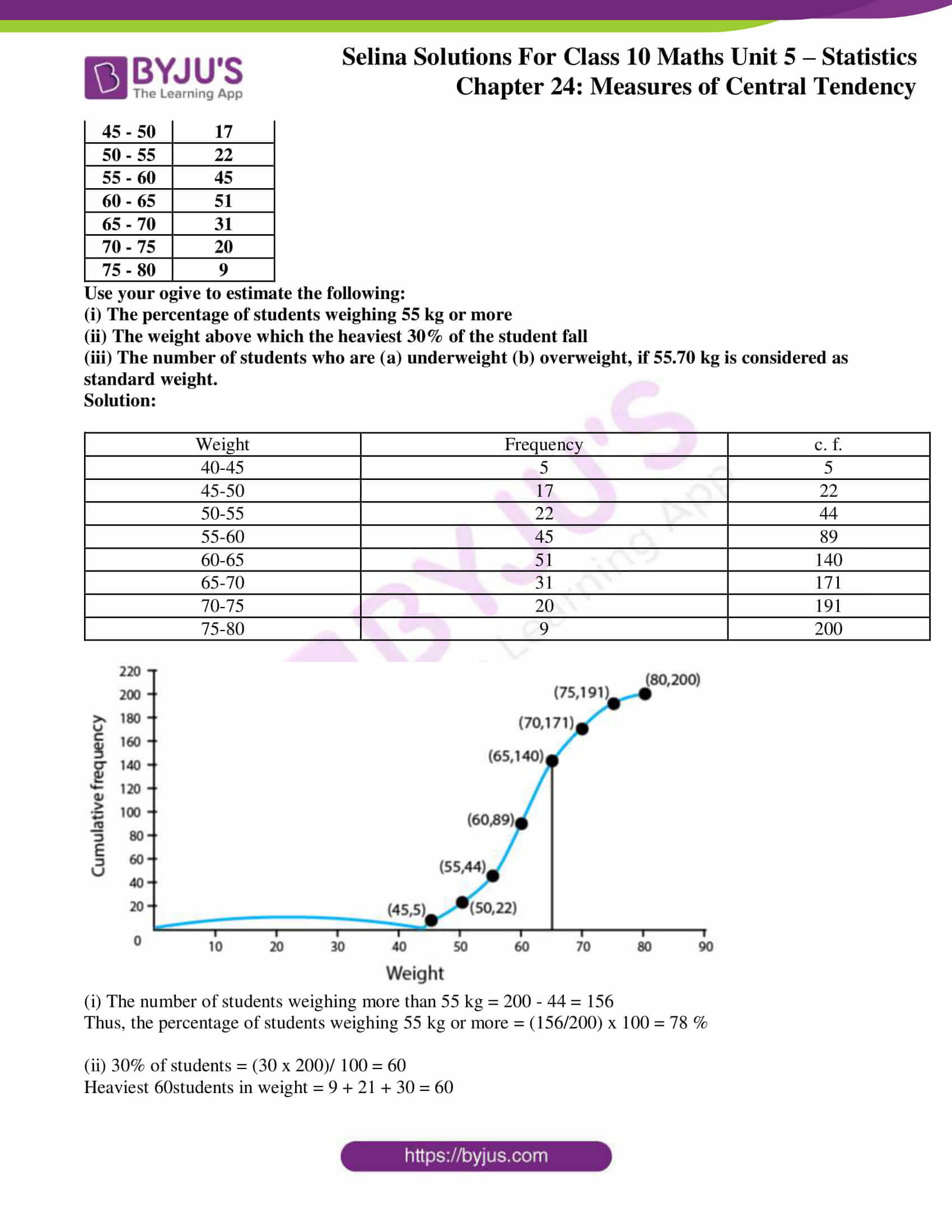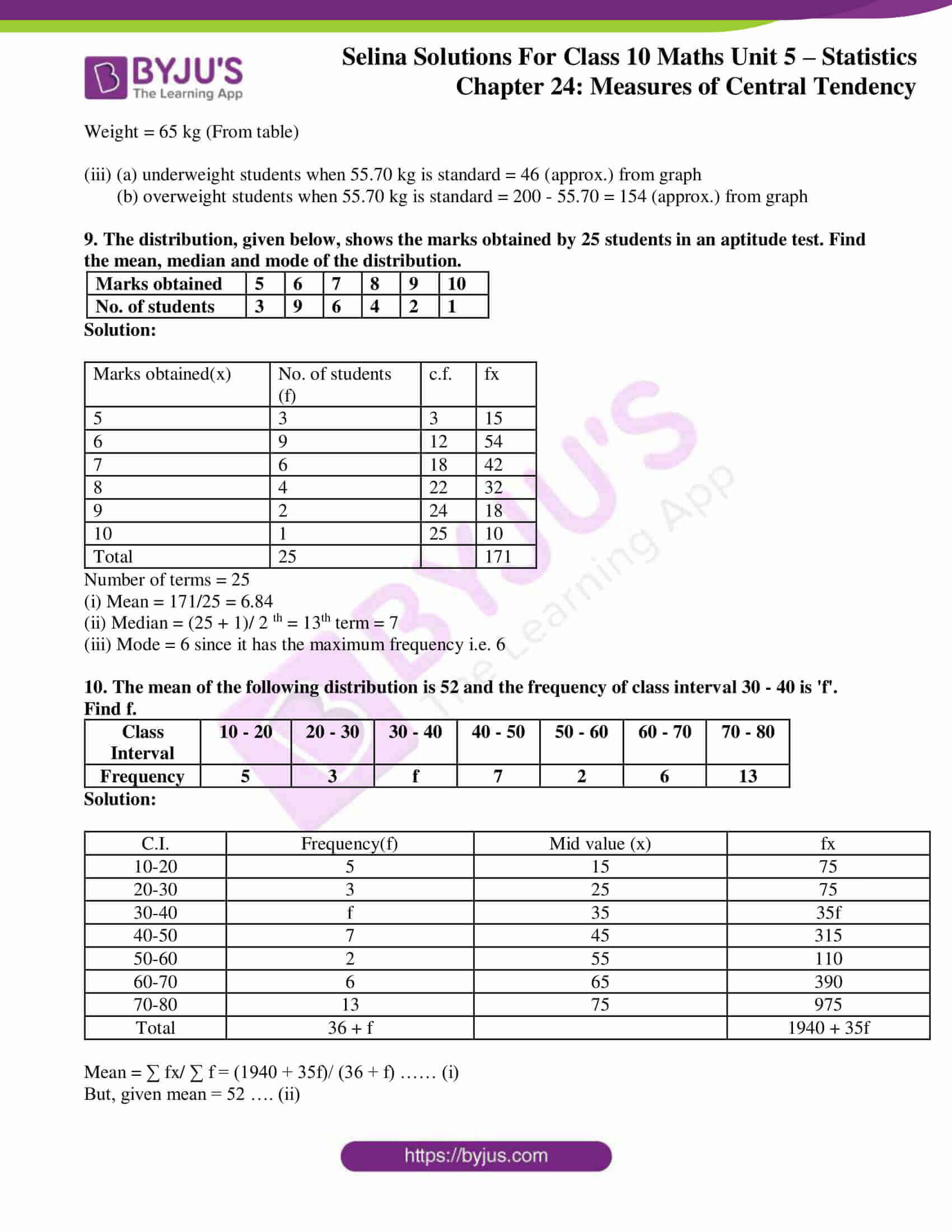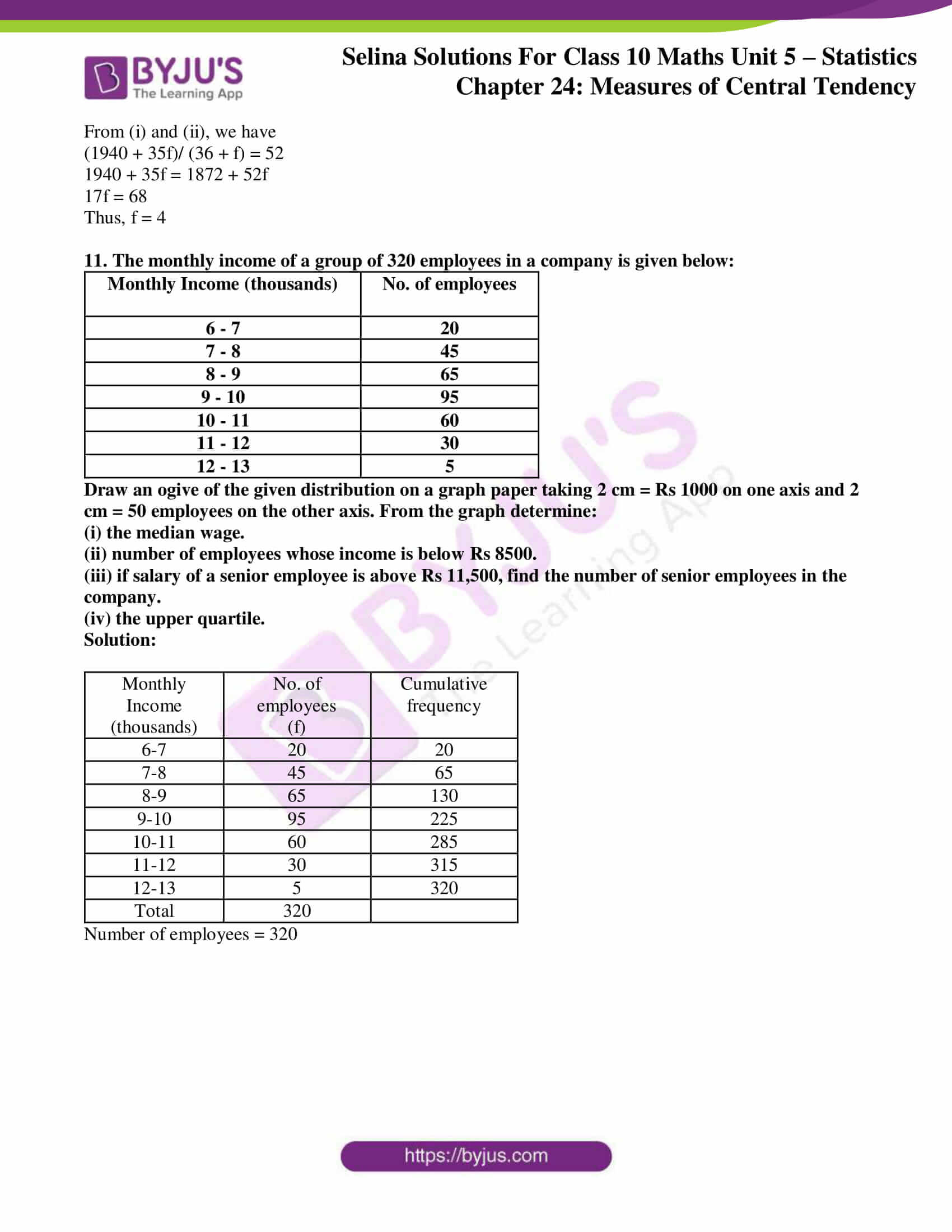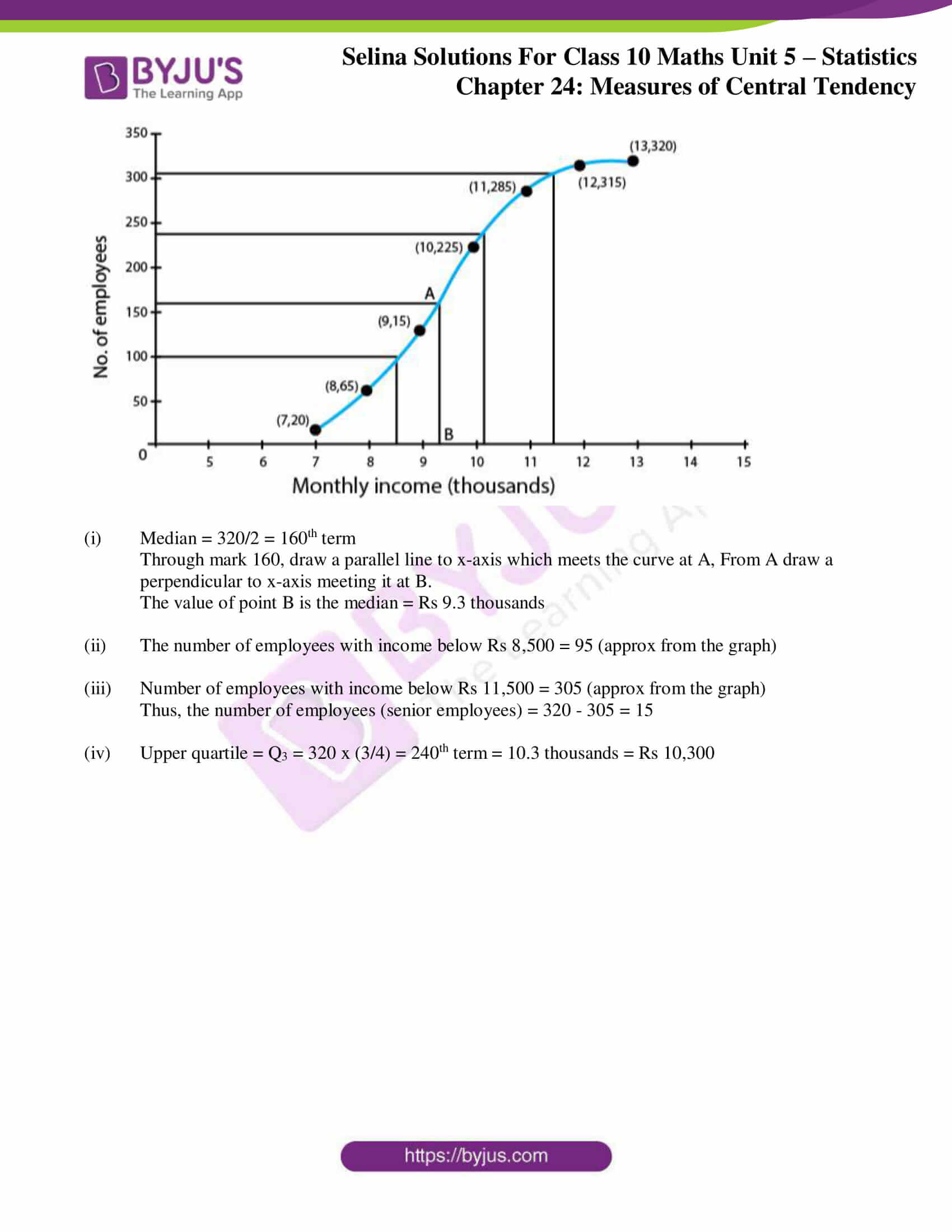### Exercises of Concise Selina Solutions Class 10 Maths Chapter 24 Measures of Central Tendency

Exercise 24(A) Solutions

Exercise 24(B) Solutions

Exercise 24(C) Solutions

Exercise 24(D) Solutions

Exercise 24(E) Solutions

## Access Selina Solutions Concise Maths Class 10 Chapter 24 Measures of Central Tendency

Exercise 24(A) Page No: 356

1. Find the mean of the following set of numbers:

(i) 6, 9, 11, 12 and 7

(ii) 11, 14, 23, 26, 10, 12, 18 and 6

Solution:

(i) By definition, we know

Mean = ∑x/ n

Here, n = 5

Thus,

Mean = (6 + 9 + 11 + 12 + 7)/ 5 = 45/5 = 9

(ii) By definition, we know

Mean = ∑x/ n

Here, n = 8

Thus,

Mean = (11 + 14 + 23 + 26 + 10 + 12 + 18 + 6)/ 8 = 120/8 = 15

2. Marks obtained (in mathematics) by 9 student are given below:

60, 67, 52, 76, 50, 51, 74, 45 and 56

(a) find the arithmetic mean

(b) if marks of each student be increased by 4; what will be the new value of arithmetic mean.

Solution:

(a) Mean = ∑x/ n

Here, n = 9

Thus,

Mean = (60 + 67 + 52 + 76 + 50 + 51 + 74 + 45 + 56)/ 9 = 531/9 = 59

(b) If the marks of each student be increased by 4 then new arithmetic mean will be = 59 + 4 = 63

3. Find the mean of the natural numbers from 3 to 12.

Solution:

The numbers between 3 to 12 are 3, 4, 5, 6, 7, 8, 9, 10, 11 and 12.

Here n = 10

Mean = ∑x/ n

= (3 + 4 + 5 + 6 + 7 + 8 + 9 + 10 + 11 + 12)/ 10 = 75/10 = 7.5

4. (a) Find the mean of 7, 11, 6, 5, and 6

(b) If each number given in (a) is diminished by 2, find the new value of mean.

Solution:

(a) Mean = ∑x/ n , here n = 5

= (7 + 11 + 6 + 5 + 6)/ 5 = 35/5 = 7

(b) If 2 is subtracted from each number, then the mean will he changed as 7 – 2 = 5

5. If the mean of 6, 4, 7, ‘a’ and 10 is 8. Find the value of ‘a’

Solution:

Given,

No. of terms (n) = 5

Mean = 8

Sum of all terms = 8 x 5 = 40 …… (i)

But, sum of numbers = 6 + 4 + 7 + a + 10 = 27 + a ….. (ii)

On equating (i) and (ii), we get

27 + a = 40

Thus, a = 13

6. The mean of the number 6, ‘y’, 7, ‘x’ and 14 is 8. Express ‘y’ in terms of ‘x’.

Solution:

Given,

No. of terms (n) = 5 and mean = 8

So, the sum of all terms = 5 x 8 = 40 ……. (i)

but sum of numbers = 6 + y + 7 + x + 14 = 27 + y + x ……. (ii)

On equating (i) and (ii), we get

27 + y + x = 40

x + y = 13

Hence, y = 13 – x

7. The ages of 40 students are given in the following table:

 Age( in yrs) 12 13 14 15 16 17 18 Frequency 2 4 6 9 8 7 4

Find the arithmetic mean.

Solution:

 Age in yrs xi Frequency (fi) fixi 12 2 24 13 4 52 14 6 84 15 9 135 16 8 128 17 7 119 18 4 72 Total 40 614

Mean = ∑fi xi/ ∑fi = 614/40 = 15.35

Exercise 24(B) Page No: 361

1. The following table gives the ages of 50 students of a class. Find the arithmetic mean of their ages.

 Age – Years 16 – 18 18 – 20 20 – 22 22- 24 24-26 No. of Students 2 7 21 17 3

Solution:

 Age in years C.I. xi Number of students (fi) xifi 16 – 18 17 2 34 18 – 20 19 7 133 20 – 22 21 21 441 22 – 24 23 17 391 24 – 26 25 3 75 Total 50 1074

Mean = ∑fi xi/ ∑fi = 1074/50 = 21.48

2. The following table gives the weekly wages of workers in a factory.

 Weekly Wages (Rs) No. of Workers 50-55 5 55-60 20 60-65 10 65-70 10 70-75 9 75-80 6 80-85 12 85-90 8

Calculate the mean by using:

(i) Direct Method

(ii) Short – Cut Method

Solution:

(i) Direct Method

 Weekly Wages (Rs) Mid-Value xi No. of Workers (fi) fixi 50-55 52.5 5 262.5 55-60 57.5 20 1150.0 60-65 62.5 10 625.0 65-70 67.5 10 675.0 70-75 72.5 9 652.5 75-80 77.5 6 465.0 80-85 82.5 12 990.0 85-90 87.5 8 700.0 Total 80 5520.00

Mean = ∑fi xi/ ∑fi = 5520/80 = 69

(ii) Short – cut method

 Weekly wages (Rs) No. of workers (fi) Mid-value xi A = 72.5 di = x – A fidi 50-55 5 52.5 -20 -100 55-60 20 57.5 -15 -300 60-65 10 62.5 -10 -100 65-70 10 67.5 -5 -50 70-75 9 A = 72.5 0 0 75-80 6 77.5 5 30 80-85 12 82.5 10 120 85-90 8 87.5 15 120 Total 80 -280

Here, A = 72.5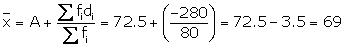3. The following are the marks obtained by 70 boys in a class test:

 Marks No. of boys 30 – 40 10 40 – 50 12 50 – 60 14 60 – 70 12 70 – 80 9 80 – 90 7 90 – 100 6

Calculate the mean by:

(i) Short – cut method

(ii) Step – deviation method

Solution:

(i) Short – cut method

 Marks No. of boys (fi) Mid-value xi A = 65 di = x – A fidi 30 – 40 10 35 -30 -300 40 – 50 12 45 -20 -240 50 – 60 14 55 -10 -140 60 – 70 12 A = 65 0 0 70 – 80 9 75 10 90 80 – 90 7 85 20 140 90 – 100 6 95 30 180 Total 70 -270

Here, A = 65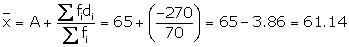(ii) Step – deviation method

 Marks No. of boys (fi) Mid-value xi A = 65 ui = (xi – A)/ h fiui 30 – 40 10 35 -3 -30 40 – 50 12 45 -2 -24 50 – 60 14 55 -1 -14 60 – 70 12 A = 65 0 0 70 – 80 9 75 1 9 80 – 90 7 85 2 14 90 – 100 6 95 3 18 Total 70 -27

Here, A = 65 and h = 10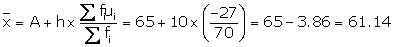4. Find mean by step – deviation method:

 C. I. 63-70 70-77 77-84 84-91 91-98 98-105 105-112 Freq 9 13 27 38 32 16 15

Solution:

 C. I. Frequency (fi) Mid-value xi A = 87.50 ui = (xi – A)/ h fiui 63 – 70 9 66.50 -3 -27 70 – 77 13 73.50 -2 -26 77 – 84 27 80.50 -1 -27 84 – 91 38 A = 87.50 0 0 91 – 98 32 94.50 1 32 98 – 105 16 101.50 2 32 105 – 112 15 108.50 3 45 Total 150 29

Here, A = 87.50 and h = 7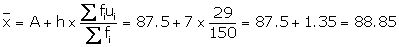5. The mean of the following frequency distribution is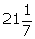. Find the value of ‘f’.

 C. I. 0 – 10 10 – 20 20 – 30 30 – 40 40 – 50 freq 8 22 31 f 2

Solution:

Given,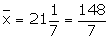C. I. frequency Mid-value (xi) fixi 0-10 8 5 40 10-20 22 15 330 20-30 31 25 775 30-40 f 35 35f 40-50 2 45 90 Total 63 + f 1235 + 35f9324 + 148f = 8645 + 245f

245f – 148f = 9324 – 8645

f = 679/97

Thus, f = 7

Exercise 24(C) Page No: 372

1. A student got the following marks in 9 questions of a question paper.

3, 5, 7, 3, 8, 0, 1, 4 and 6.

Find the median of these marks.

Solution:

Arranging the given data in descending order:

8, 7, 6, 5, 4, 3, 3, 1, 0

Clearly, the middle term is 4 which is the 5th term.

Hence, median = 4

2. The weights (in kg) of 10 students of a class are given below:

21, 28.5, 20.5, 24, 25.5, 22, 27.5, 28, 21 and 24.

Find the median of their weights.

Solution:

Arranging the given data in descending order:

28.5, 28, 27.5, 25.5, 24, 24, 22, 21, 21, 20.5

It’s seen that,

The middle terms are 24 and 24, 5th and 6th terms

Thus,

Median = (24 + 24)/ 2 = 48/2 = 24

3. The marks obtained by 19 students of a class are given below:

27, 36, 22, 31, 25, 26, 33, 24, 37, 32, 29, 28, 36, 35, 27, 26, 32, 35 and 28. Find:

(i) median (ii) lower quartile

(iii) upper quartile (iv) interquartile range

Solution:

Arranging in ascending order:

22, 24, 25, 26, 26, 27, 27, 28, 28, 29, 21, 32, 32, 33, 35, 35, 36, 36, 37

(i) The middle term is 10th term i.e. 29

Hence, median = 29

(ii) Lower quartile(iii) Upper quartile =(iv) Interquartile range = q3 – q1 =35 – 26 = 9

4. From the following data, find:

(i) Median

(ii) Upper quartile

(iii) Inter-quartile range

25, 10, 40, 88, 45, 60, 77, 36, 18, 95, 56, 65, 7, 0, 38 and 83

Solution:

Arranging the given data in ascending order, we have:

0, 7, 10, 18, 25, 36, 38, 40, 45, 56, 60, 65, 77, 83, 88, 95

(i) Median is the mean of 8th and 9th term

Thus, median = (40 + 45)/ 2 = 85/2 = 42.5

(ii) Upper quartile =(iii) Interquartile range is given by,

q1 = 16th/4 term = 18; q3 = 65

Interquartile range = q3 – q1

Thus,

q3 – q1 = 65 – 18 = 47

5. The ages of 37 students in a class are given in the following table:

 Age (in years) 11 12 13 14 15 16 Frequency 2 4 6 10 8 7

Find the median.

Solution:

 Age (in years) Frequency Cumulative Frequency 11 2 2 12 4 6 13 6 12 14 10 22 15 8 30 16 7 37

Number of terms (n) = 37

Median =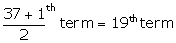And, the 19th term is 14

Therefore, the median = 14

Exercise 24(D) Page No: 374

1. Find the mode of the following data:

(i) 7, 9, 8, 7, 7, 6, 8, 10, 7 and 6

(ii) 9, 11, 8, 11, 16, 9, 11, 5, 3, 11, 17 and 8

Solution:

(i) It’s seen that 7 occurs 4 times in the given data.

Hence, mode = 7

(ii) Mode = 11

As 11 occurs 4 times in the given data.

2. The following table shows the frequency distribution of heights of 50 boys:

 Height (cm) 120 121 122 123 124 Frequency 5 8 18 10 9

Find the mode of heights.

Solution:

Clearly,

Mode is 122 cm because it has occurred the maximum number of times.

i.e. frequency is 18.

3. Find the mode of following data, using a histogram:

 Class 0-10 10-20 20-30 30-40 40-50 Frequency 5 12 20 9 4

Solution: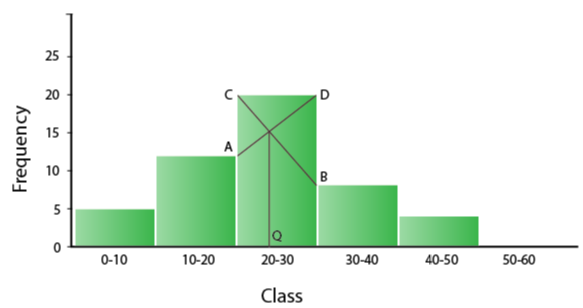Clearly,

Mode is in 20-30, because in this class there are 20 frequencies.

4. The following table shows the expenditure of 60 boys on books. Find the mode of their expenditure:

 Expenditure (Rs) No. of students 20-25 4 25-30 7 30-35 23 35-40 18 40-45 6 45-50 2

Solution: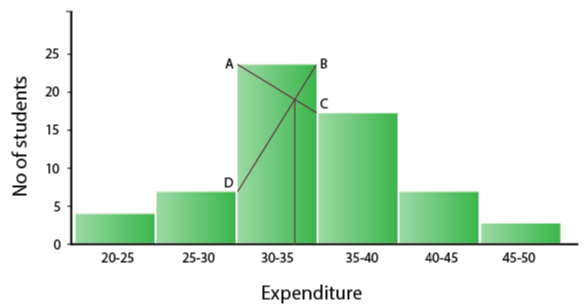Clearly,

Mode is in 30-35 because it has the maximum frequency.

Exercise 24(E) Page No: 375

1. The following distribution represents the height of 160 students of a school.

 Height (in cm) No. of Students 140 – 145 12 145 – 150 20 150 – 155 30 155 – 160 38 160 – 165 24 165 – 170 16 170 – 175 12 175 – 180 8

Draw an ogive for the given distribution taking 2 cm = 5 cm of height on one axis and 2 cm = 20 students on the other axis. Using the graph, determine:

i. The median height.

ii. The interquartile range.

iii. The number of students whose height is above 172 cm.

Solution:

 Height (in cm) No. of Students Cumulative frequency 140 – 145 12 12 145 – 150 20 32 150 – 155 30 62 155 – 160 38 100 160 – 165 24 124 165 – 170 16 140 170 – 175 12 152 175 – 180 8 160 N = 160

Now, let’s draw an ogive taking height of student along x-axis and cumulative frequency along y-axis.(i) So,

Median = 160/2 = 80th term

Through mark for 80, draw a parallel line to x-axis which meets the curve; then from the curve draw a vertical line which meets the x-axis at the mark of 157.5.

(ii) As, the number of terms = 160

Lower quartile (Q1) = (160/4) = 40th term = 152

Upper quartile (Q3) = (3 x 160/4) = 120th term = 164

Inner Quartile range = Q3 – Q1

= 164 – 152

= 12

(iii) Through mark for 172 on x-axis, draw a vertical line which meets the curve; then from the curve draw a horizontal line which meets the y-axis at the mark of 145.

Now,

The number of students whose height is above 172 cm

= 160 – 144 = 16

2. Draw ogive for the data given below and from the graph determine: (i) the median marks.

(ii) the number of students who obtained more than 75% marks.

 Marks 10 – 19 20 -29 30 – 39 40 – 49 50 – 59 60 – 69 70 – 79 80 – 89 90 – 99 No. of students 14 16 22 26 18 11 6 4 3

Solution:

 Marks No. of students Cumulative frequency 9.5 – 19.5 14 14 19.5 – 29.5 16 30 29.5 – 39.5 22 52 39.5 – 49.5 26 78 49.5 – 59.5 18 96 59.5 – 69.5 11 107 69.5 – 79.5 6 113 79.5 – 89.5 4 117 89.5 – 99.5 3 120

Scale:

1cm = 10 marks on X axis

1cm = 20 students on Y axis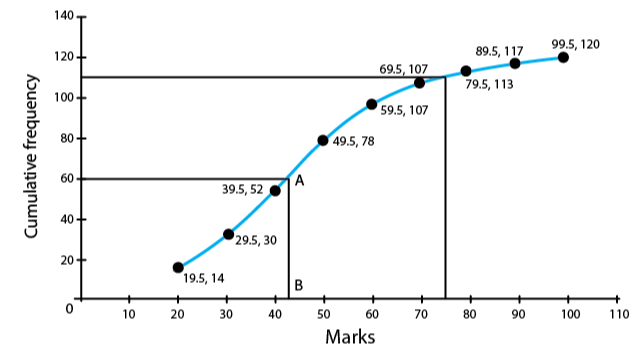(i) So, the median = 120/ 2 = 60th term

Through mark 60, draw a parallel line to x-axis which meets the curve at A. From A, draw a perpendicular to x-axis meeting it at B.

The value of point B is the median = 43

(ii) Total marks = 100

75% of total marks = 75/100 x 100 = 75 marks

Hence, the number of students getting more than 75% marks = 120 – 111 = 9 students.

3. The mean of 1, 7, 5, 3, 4 and 4 is m. The numbers 3, 2, 4, 2, 3, 3 and p have mean m – 1 and median q. Find p and q.

Solution:

Mean of 1, 7, 5, 3, 4 and 4 = (1 + 7 + 5 + 3 + 4 + 4)/ 6 = 24/6 = 4

So, m = 4

Now, given that

The mean of 3, 2, 4, 2, 3, 3 and p = m -1 = 4 – 1 = 3

Thus, 17 + p = 3 x n …. ,where n = 7

17 + p = 21

p = 4

Arranging the terms in ascending order, we have:

2, 2, 3, 3, 3, 3, 4, 4

Mean = 4th term = 3

Hence, q = 3

4. In a malaria epidemic, the number of cases diagnosed were as follows:

 Date (July) 1 2 3 4 5 6 7 8 9 10 11 12 Number 5 12 20 27 46 30 31 18 11 5 0 1

On what days do the mode and upper and lower quartiles occur?

Solution:

 Date Number C.f. 1 5 5 2 12 17 3 20 37 4 27 64 5 46 110 6 30 140 7 31 171 8 18 189 9 11 200 10 5 205 11 0 205 12 1 206

(i) Mode = 5th July as it has maximum frequencies.

(ii) Total number of terms = 206

Upper quartile = 206 x (3/4) = 154.5th = 7th July

Lower quartile = 206 x (1/4) = 51.5th = 4th July

5. The income of the parents of 100 students in a class in a certain university are tabulated below.

 Income (in thousand Rs) 0 – 8 8 – 16 16 – 24 24 – 32 32 – 40 No. of students 8 35 35 14 8

(i) Draw a cumulative frequency curve to estimate the median income.

(ii) If 15% of the students are given freeships on the basis of the basis of the income of their parents, find the annual income of parents, below which the freeships will be awarded.

(iii) Calculate the Arithmetic mean.

Solution: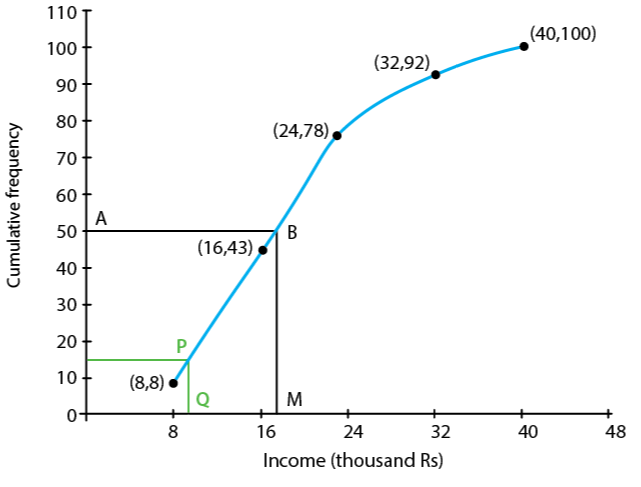(i) Cumulative Frequency Curve

 Income (in thousand Rs.) No. of students f Cumulative Frequency Class mark x fx 0 – 8 8 8 4 32 8 – 16 35 43 12 420 16 – 24 35 78 20 700 24 – 32 14 92 28 392 32 – 40 8 100 36 288 ∑f = 100 ∑ fx = 1832

We plot the points (8, 8), (16, 43), (24, 78), (32, 92) and (40, 100) to get the curve as follows:

Here, N = 100

N/2 = 50

At y = 50, affix A.

Through A, draw a horizontal line meeting the curve at B.

Through B, a vertical line is drawn which meets OX at M.

OM = 17.6 units

Hence, median income = 17.6 thousands

(ii) 15% of 100 students = (15 x 100)/ 100 = 15

From c.f. 15, draw a horizontal line which intersects the curve at P.

From P, draw a perpendicular to x – axis meeting it at Q which is equal to 9.6

Thus, freeship will be awarded to students provided annual income of their parents is upto 9.6 thousands.

(ii) Mean = ∑ fx/ ∑ f = 1832/100 = 18.32

6. The marks of 20 students in a test were as follows:

2, 6, 8, 9, 10, 11, 11, 12, 13, 13, 14, 14, 15, 15, 15, 16, 16, 18, 19 and 20.

Calculate:

(i) the mean (ii) the median (iii) the mode

Solution:

Arranging the terms in ascending order:

2, 6, 8, 9, 10, 11, 11, 12, 13, 13, 14, 14, 15, 15, 15, 16, 16, 18, 19, 20

Number of terms = 20

∑ x = 2 + 6 + 8 + 9 + 11 + 11 + 12 + 13 + 13 + 14 + 14 + 15 + 15 + 15 + 15 + 16 + 16 + 18 + 19 + 20 = 257

(i) Mean = ∑x/ ∑n = 257/ 20 = 12.85

(ii) Median = (10th term + 11th term)/ 2 = (13 + 14)/ 2 = 27/ 2 = 13.5

(iii) Mode = 15 since it has maximum frequencies i.e. 3

7. The marks obtained by 120 students in a mathematics test is given below:

 Marks No. of students 0-10 5 10-20 9 20-30 16 30-40 22 40-50 26 50-60 18 60-70 11 70-80 6 80-90 4 90-100 3

Draw an ogive for the given distribution on a graph sheet. Use a suitable scale for your ogive. Use your ogive to estimate:

(i) the median

(ii) the number of students who obtained more than 75% in test.

(iii) the number of students who did not pass in the test if the pass percentage was 40.

(iv) the lower quartile

Solution:

 Marks No. of students c.f. 0-10 5 5 10-20 9 14 20-30 16 30 30-40 22 52 40-50 26 78 50-60 18 96 60-70 11 107 70-80 6 113 80-90 4 117 90-100 3 120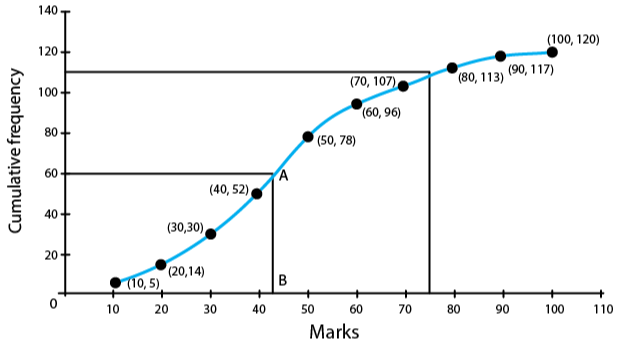(i)  Median = (120 + 1)/ 2 = 60.5th term

Through mark 60.5, draw a parallel line to x-axis which meets the curve at A. From A draw a perpendicular to x-axis meeting it at B.

Then, the value of point B is the median = 43

(ii) Number of students who obtained up to 75% marks in the test = 110

Number of students who obtained more than 75% marks in the test = 120 – 110 = 10

(iii) Number of students who obtained less than 40% marks in the test = 52 (from the graph; x = 40, y = 52)

(iv) Lower quartile = Q1 = 120 x (1/4) = 30th term = 30

8. Using a graph paper, draw an ogive for the following distribution which shows a record of the width in kilograms of 200 students.

 Weight Frequency 40 – 45 5 45 – 50 17 50 – 55 22 55 – 60 45 60 – 65 51 65 – 70 31 70 – 75 20 75 – 80 9

Use your ogive to estimate the following:

(i) The percentage of students weighing 55 kg or more

(ii) The weight above which the heaviest 30% of the student fall

(iii) The number of students who are (a) underweight (b) overweight, if 55.70 kg is considered as standard weight.

Solution:

 Weight Frequency c. f. 40-45 5 5 45-50 17 22 50-55 22 44 55-60 45 89 60-65 51 140 65-70 31 171 70-75 20 191 75-80 9 200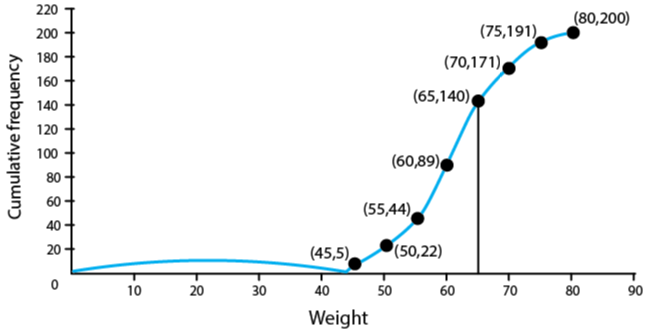(i) The number of students weighing more than 55 kg = 200 – 44 = 156

Thus, the percentage of students weighing 55 kg or more = (156/200) x 100 = 78 %

(ii) 30% of students = (30 x 200)/ 100 = 60

Heaviest 60students in weight = 9 + 21 + 30 = 60

Weight = 65 kg (From table)

(iii) (a) underweight students when 55.70 kg is standard = 46 (approx.) from graph

(b) overweight students when 55.70 kg is standard = 200 – 55.70 = 154 (approx.) from graph

9. The distribution, given below, shows the marks obtained by 25 students in an aptitude test. Find the mean, median and mode of the distribution.

 Marks obtained 5 6 7 8 9 10 No. of students 3 9 6 4 2 1

Solution:

 Marks obtained(x) No. of students (f) c.f. fx 5 3 3 15 6 9 12 54 7 6 18 42 8 4 22 32 9 2 24 18 10 1 25 10 Total 25 171

Number of terms = 25

(i) Mean = 171/25 = 6.84

(ii) Median = (25 + 1)/ 2 th = 13th term = 7

(iii) Mode = 6 since it has the maximum frequency i.e. 6

10. The mean of the following distribution is 52 and the frequency of class interval 30 – 40 is ‘f’. Find f.

 Class Interval 10 – 20 20 – 30 30 – 40 40 – 50 50 – 60 60 – 70 70 – 80 Frequency 5 3 f 7 2 6 13

Solution:

 C.I. Frequency(f) Mid value (x) fx 10-20 5 15 75 20-30 3 25 75 30-40 f 35 35f 40-50 7 45 315 50-60 2 55 110 60-70 6 65 390 70-80 13 75 975 Total 36 + f 1940 + 35f

Mean = ∑ fx/ ∑ f = (1940 + 35f)/ (36 + f) …… (i)

But, given mean = 52 …. (ii)

From (i) and (ii), we have

(1940 + 35f)/ (36 + f) = 52

1940 + 35f = 1872 + 52f

17f = 68

Thus, f = 4

11. The monthly income of a group of 320 employees in a company is given below:

 Monthly Income (thousands) No. of employees 6 – 7 20 7 – 8 45 8 – 9 65 9 – 10 95 10 – 11 60 11 – 12 30 12 – 13 5

Draw an ogive of the given distribution on a graph paper taking 2 cm = Rs 1000 on one axis and 2 cm = 50 employees on the other axis. From the graph determine:

(i) the median wage.

(ii) number of employees whose income is below Rs 8500.

(iii) if salary of a senior employee is above Rs 11,500, find the number of senior employees in the company.

(iv) the upper quartile.

Solution:

 Monthly Income (thousands) No. of employees (f) Cumulative frequency 6-7 20 20 7-8 45 65 8-9 65 130 9-10 95 225 10-11 60 285 11-12 30 315 12-13 5 320 Total 320

Number of employees = 320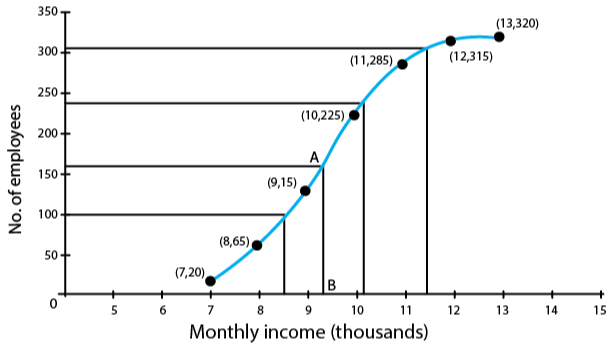(i) Median = 320/2 = 160th term

Through mark 160, draw a parallel line to x-axis which meets the curve at A, From A draw a perpendicular to x-axis meeting it at B.

The value of point B is the median = Rs 9.3 thousands

(ii) The number of employees with income below Rs 8,500 = 95 (approx from the graph)

(iii) Number of employees with income below Rs 11,500 = 305 (approx from the graph)

Thus, the number of employees (senior employees) = 320 – 305 = 15

(iv) Upper quartile = Q3 = 320 x (3/4) = 240th term = 10.3 thousands = Rs 10,300

The given solutions are as per the 2019-20 Concise Selina textbook. The Selina Solutions for the academic year 2020-21 will be updated soon.